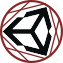#Uninomicon

Documenting the dark corners of the Unity Engine.

navmeshagent

# NavMeshAgent

This function does only respond with the current remaining distance if the path is straight. It will thus return null or infinite instead of the correct distance (as defined on the Unity documentation). There is a way to calculate the real distance by combining all calculated paths but keep in mind that this is performance heavy.

First example on stack-overflow:

```public static class ExtensionMethods
{
public static float GetPathRemainingDistance(this NavMeshAgent navMeshAgent)
{
if (navMeshAgent.pathPending ||
navMeshAgent.pathStatus == NavMeshPathStatus.PathInvalid ||
navMeshAgent.path.corners.Length == 0)
return -1f;

float distance = 0.0f;
for (int i = 0; i < navMeshAgent.path.corners.Length - 1; ++i)
{
distance += Vector3.Distance(navMeshAgent.path.corners[i], navMeshAgent.path.corners[i + 1]);
}

return distance;
}
}```